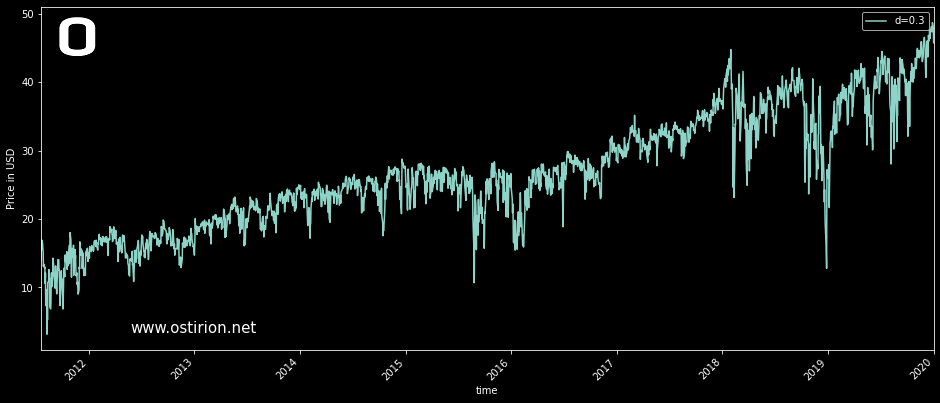top of page
Search

# Stock Price Fractional Differentiation: Best Fraction Finder

Updated: Mar 22, 2021

This post extends the code for our interpretation of the fractional differentiation calculation methods presented in Marcos Lopez de Prado. 2018. Advances in Financial Machine Learning (1st. ed.). Wiley Publishing. Our initial steps are in this post, where we implement the standard factional differentiation procedure. In this post, our next step is to implement a fixed-width window method. As the data window expands in our previous method, either the oldest observations dominate the memory of the series and mask more recent changes, or the weighting limit will cut-off a significant part of the data out of the analysis to capture those recent changes. The fixed-width window method alleviates this problem using the same weight vector for each data point, truncated at a threshold value.

First of all, we have to redefine the weighting function to compute the weights until the point in which they are below the threshold, when the calculation stops, and we obtain a fixed-length array of weights:

```def compute_weights_fixed_window(d: float,
threshold: float=1e-5) -> pd.DataFrame:
'''
Compute the weights of individual data points for fractional
differentiation with fixed window:
Args:
d (float): Fractional differentiation value.
threshold (float): Minimum weight to calculate.

Returns:
pd.DataFrame: Dataframe containing the weights for each point.
'''
w = [1.0]
k = 1while True:
v = -w[-1]/k*(d-k+1)
if abs(v) < threshold:
break
w.append(v)
k += 1

w = np.array(w[::-1]).reshape(-1, 1)
return pd.DataFrame(w)
```

The function still returns a pandas dataframe with the weights; it will have the same length for each of the computed differences for all possible values of d. Using these weights, We can compute the series of fractional differences:

```def fixed_window_fracc_diff(df: pd.DataFrame,
d: float,
threshold: float=1e-5) -> pd.DataFrame:
'''
Compute the d fractional difference of the series with a fixed
width window.
Args:
df (pd.DataFrame): Dataframe with series to be differentiated.
d (float): Order of differentiation.
threshold (float): threshold value to drop non-significant
weights.
Returns:
pd.DataFrame: Dataframe containing differentiated series.
'''

w = compute_weights_fixed_window(d, threshold)
l = len(w)
results = {}
names = df.columns
for name in names:
series_f = df[name].fillna(method='ffill').dropna()

if l > series_f.shape:
return standard_frac_diff(df, d, threshold)
r = range(l, series_f.shape)
df_ = pd.Series(index=r)

for idx in r:
if not np.isfinite(df[name].iloc[idx]):
continue
results[idx] = np.dot(w.iloc[-(idx):, :].T,
series_f.iloc[idx-l:idx])

result = pd.DataFrame(pd.Series(results), columns=['Frac_diff'])
result.set_index(df[l:].index, inplace=True)

return result```

When our fixed window is exhausted in this implementation, the calculation defaults to a standard fractional difference computation, this is useful when finding the optimal value for d that produces a stationary series with minimum information loss. If the window is exhausted, the fixed-width window method will return an empty series, as there is no solution for this method. Using the standard method for those lower values of d yields time series that are still useful. We will illustrate this effect in future posts by mixing common financial instruments that require different differentiation levels for stationarity (using possibly SPY and VIX).

Plotting the results for the 0 to 1 linear space for values of d generates the following familiar plot for SPY history:For comparison purposes, this is the same figure for the standard expanding window fractional differentiation, showing much less information for lower values of differentiation, where we may find the most useful data for machine learning features:With this flexible standard-fixed-width model, we can safely search for the most useful fractionally differentiated series, that with the lowest value for d that yields a stationary series. We are going to check for stationarity with the Augmented Dickey-Fuller test (see here). The test will be applied across the complete 0-1 space; the linspace argument can control the resolution of the search space:

```def find_stat_series(df: pd.DataFrame,
threshold: float=0.0001,
diffs: np.linspace=np.linspace(0.05, 0.95, 19),
p_value: float=0.05) -> pd.DataFrame:
'''
Find the series that passes the adf test at the given p_value.
The time series must be a single column dataframe.
Args:
df (pd.DataFrame): Dataframe with series to be differentiated.
threshold (float): threshold value to drop non-significant weights.
diffs (np.linspace): Space for candidate d values.
p_value (float): ADF test p-value limit for rejection of null
hypothesis.
Returns:
pd.DataFrame: Dataframe containing differentiated series. This
series is stationary and maintains maximum memory information.
'''
for diff in diffs:
if diff == 0:
continue
s = fixed_window_fracc_diff(df, diff, threshold)
s.columns = ['d='+str(diff)]
return s```

The function returns the "best" fractionally differentiated time series. For historical SPY daily close data (2010 to 2020), this is the resulting dataframe. Note that the corresponding value for d is in the column name and could be "regexed" out if it needs to be accessed:In this particular case, the earliest fractional differentiated series that passes the ADF test at a p-value of 0.05 is 0.3, quite early in the differentiation process. The shape of this series is this:The series is ready to be used as a feature for machine learning models as it captures the highest possible amount of information while behaving well statistically speaking. We are adding this code to the Fractio repository here.

Information in ostirion.net does not constitute financial advice; we do not hold positions in any of the companies or assets that we mention in our posts at the time of posting. If you require quantitative model development, deployment, verification, or validation, do not hesitate and contact us. We will also be glad to help you with your machine learning or artificial intelligence challenges when applied to asset management, trading, or risk evaluations.

On the cover for this post: Nova fractal with constant 3,0,0,-3 polynomial terms made with this online fractal generator.

The functions used in this post, as part of a notebook: